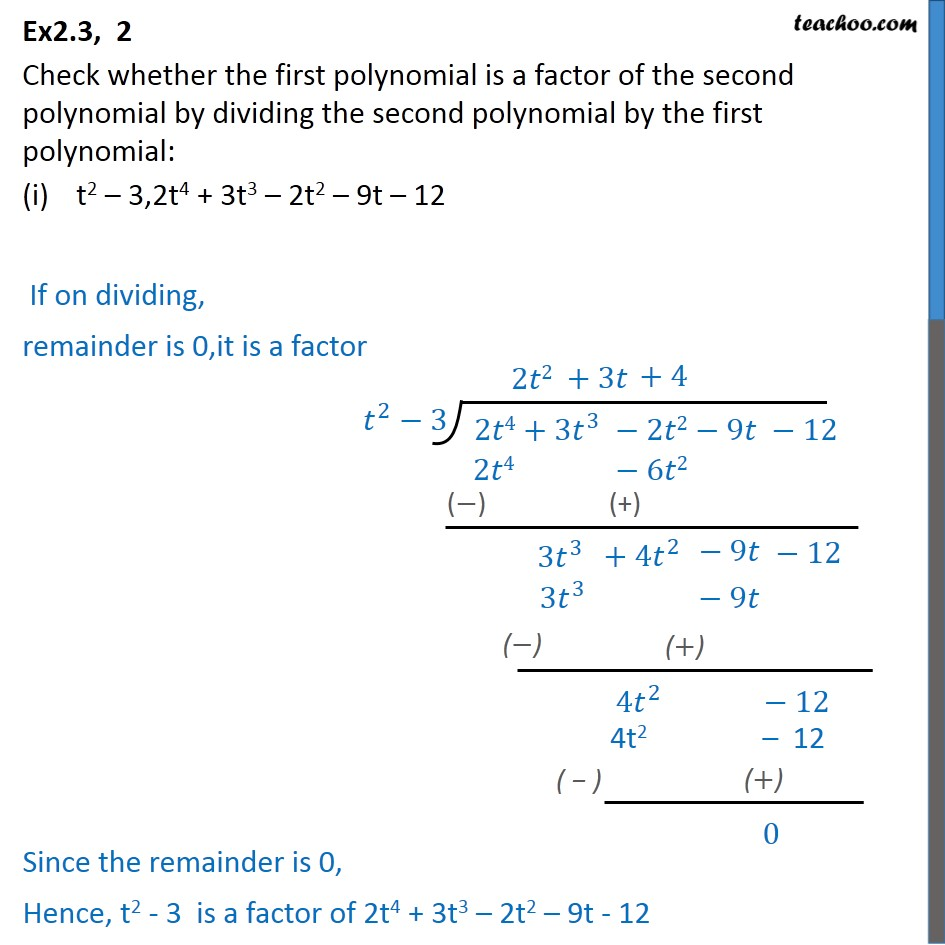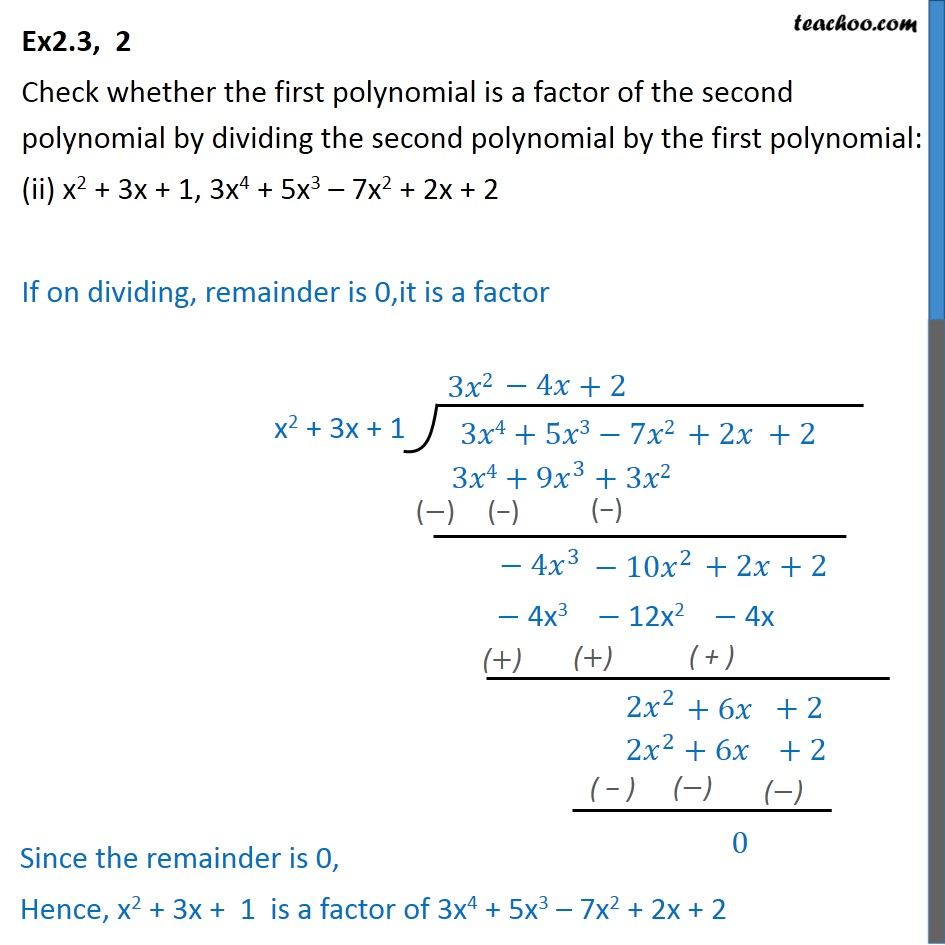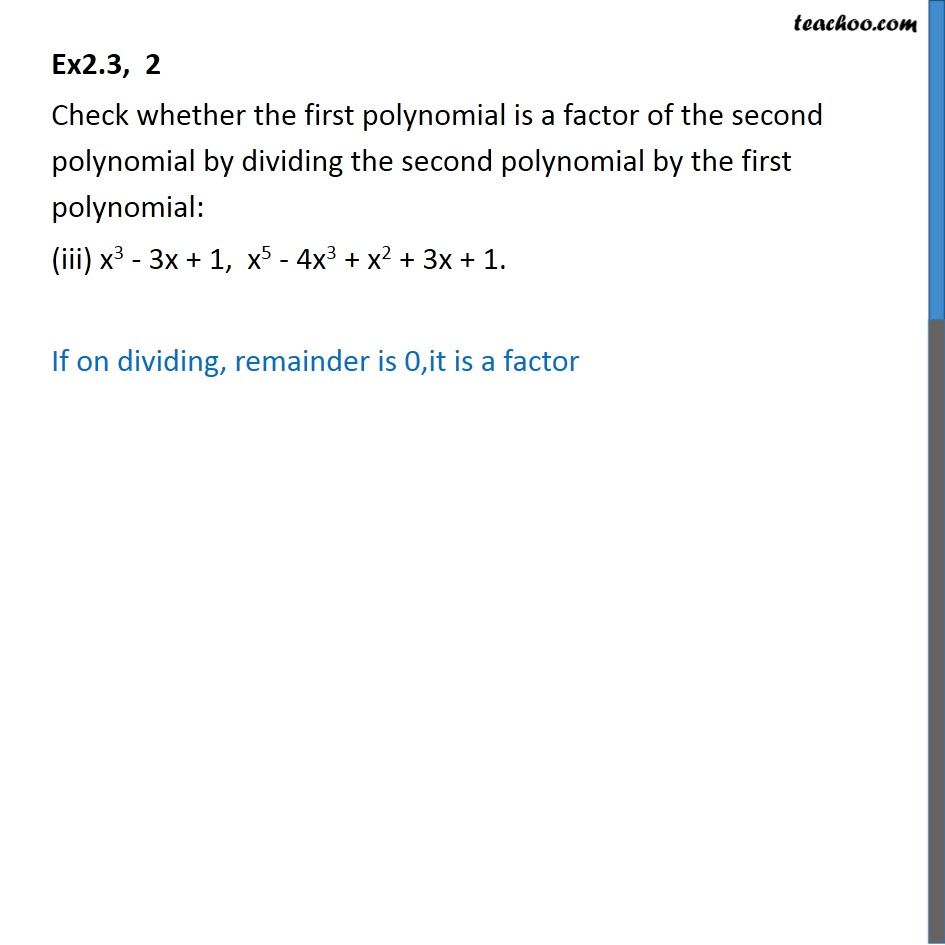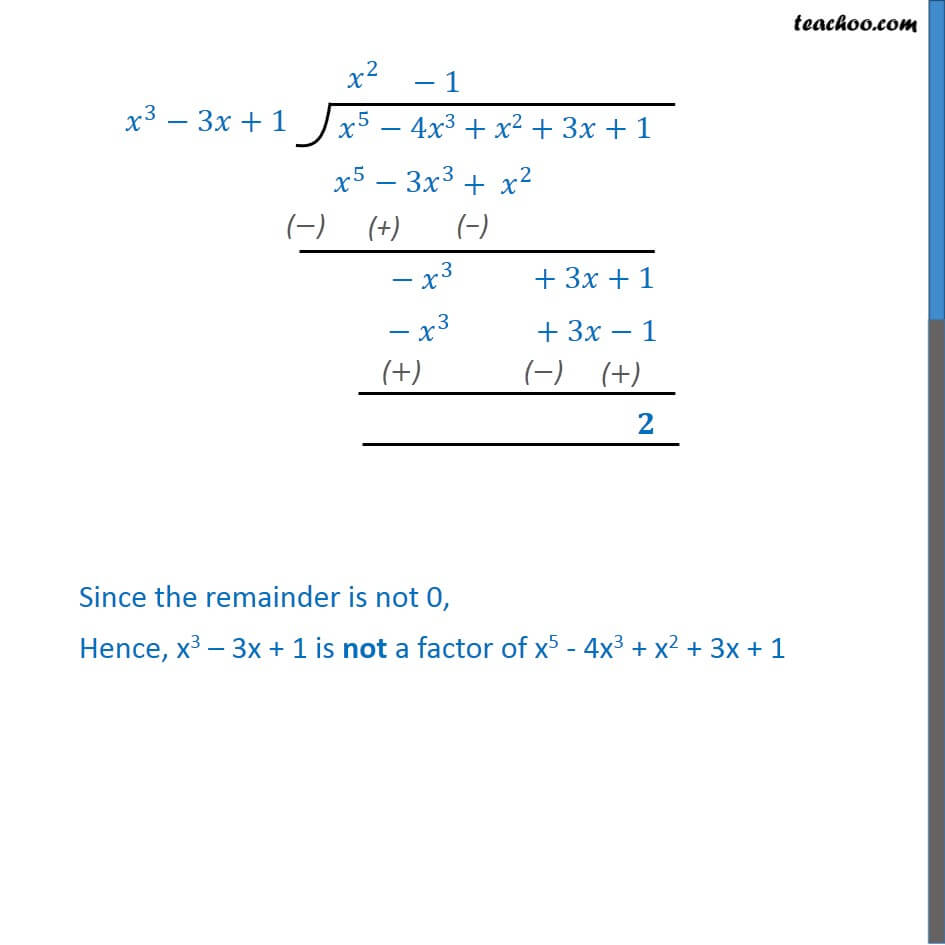Learn Polynomials - Start to Finish - FREE. Check - Polynomials Class 101. Chapter 2 Class 10 Polynomials
2. Serial order wise
3. Ex 2.3

Transcript

Ex2.3, 2 Check whether the first polynomial is a factor of the second polynomial by dividing the second polynomial by the first polynomial: t2 3,2t4 + 3t3 2t2 9t 12 If on dividing, remainder is 0,it is a factor Ex2.3, 2 Check whether the first polynomial is a factor of the second polynomial by dividing the second polynomial by the first polynomial: (ii) x2 + 3x + 1, 3x4 + 5x3 7x2 + 2x + 2 If on dividing, remainder is 0,it is a factor Ex2.3, 2 Check whether the first polynomial is a factor of the second polynomial by dividing the second polynomial by the first polynomial: (iii) x3 - 3x + 1, x5 - 4x3 + x2 + 3x + 1. If on dividing, remainder is 0,it is a factor Since the remainder is not 0, Hence, x3 3x + 1 is not a factor of x5 - 4x3 + x2 + 3x + 1

Ex 2.3

Chapter 2 Class 10 Polynomials
Serial order wise